## Segment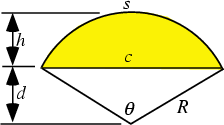A portion of a Circle whose upper boundary is a circular Arc and whose lower boundary is a Chord making a Central Angleradians (180°), illustrated above as the shaded region. Letbe the radius of the Circle,the Chord length,the Arc Length,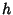the height of the arced portion, andthe height of the triangular portion. Then(1)(2)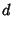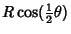(3)(4)(5)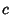(6)(7)(8)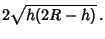(9)

The Angle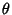obeys the relationships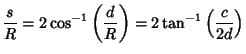(10)

The Area of the segment is then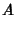(11)(12)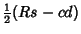(13)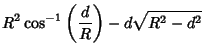(14)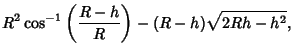(15)

where the formula for the Isosceles Triangle in terms of the Vertex angle has been used (Beyer 1987).

See also Chord, Circle-Circle Intersection, Cylindrical Segment, Lens, Parabolic Segment, Sagitta, Sector, Spherical Segment

References

Beyer, W. H. (Ed.) CRC Standard Mathematical Tables, 28th ed. Boca Raton, FL: CRC Press, p. 125, 1987.